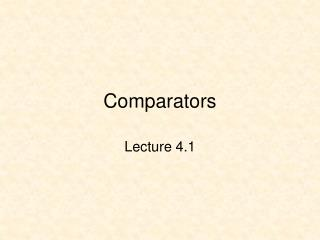# Comparators - PowerPoint PPT PresentationDownload PresentationComparators

Download Presentation## Comparators

- - - - - - - - - - - - - - - - - - - - - - - - - - - E N D - - - - - - - - - - - - - - - - - - - - - - - - - - -
##### Presentation Transcript

1. Comparators Lecture 4.1

2. Comparators Recall that an XNOR gate can be used as an equality detector XNOR X if X = Y then Z <= '1'; else Z <= '0'; end if; Z Y Z = !(X \$ Y) Z = X xnor Y Z = ~(X @ Y) X Y Z 0 0 1 0 1 0 1 0 0 1 1 1

3. 4-Bit Equality Comparator A: in STD_LOGIC_VECTOR(3 downto 0); B: in STD_LOGIC_VECTOR(3 downto 0); A_EQ_B: out STD_LOGIC;

4. library IEEE; use IEEE.STD_LOGIC_1164.ALL; use IEEE.STD_LOGIC_ARITH.ALL; use IEEE.STD_LOGIC_UNSIGNED.ALL; entity eqdet4 is Port ( A : in std_logic_vector(3 downto 0); B : in std_logic_vector(3 downto 0); A_EQ_B : out std_logic); end eqdet4; architecture Behavioral of eqdet4 is signal C: std_logic_vector(3 downto 0); begin C <= A xnor B; A_EQ_B <= C0 and C1 and C2 and C3; end Behavioral;

5. comp A_EQ_B A(n-1:0) A_GT_B A_LT_B B(n-1:0) A_UGT_B A_ULT_B Comparators A, B signed A, B unsigned Signed: 2's complement signed numbers

6. -- Comparator for unsigned and signed numbers library IEEE; use IEEE.std_logic_1164.all; use IEEE.std_logic_arith.all; use IEEE.std_logic_unsigned.all; entity comp is generic(width:positive); port ( A: in STD_LOGIC_VECTOR(width-1 downto 0); B: in STD_LOGIC_VECTOR(width-1 downto 0); A_EQ_B: out STD_LOGIC; A_GT_B: out STD_LOGIC; A_LT_B: out STD_LOGIC; A_ULT_B: out STD_LOGIC; A_UGT_B: out STD_LOGIC ); end comp; comp A_EQ_B A(n-1:0) A_GT_B A_LT_B B(n-1:0) A_UGT_B A_ULT_B

7. architecture comp_arch of comp is begin CMP: process(A,B) variable AVS, BVS: signed(width-1 downto 0); begin for i in 0 to width-1 loop AVS(i) := A(i); BVS(i) := B(i); end loop; A_EQ_B <= '0'; A_GT_B <= '0'; A_LT_B <= '0'; A_ULT_B <= '0'; A_UGT_B <= '0'; if (A = B) then A_EQ_B <= '1'; end if; if (AVS > BVS) then A_GT_B <= '1'; end if; if (AVS < BVS) then A_LT_B <= '1'; end if; if (A > B) then A_UGT_B <= '1'; end if; if (A < B) then A_ULT_B <= '1'; end if; end process CMP; end comp_arch; comp A_EQ_B A(n-1:0) A_GT_B A_LT_B B(n-1:0) A_UGT_B A_ULT_B Note: All outputs must be assigned some value. The last signal assignment in a process is the value assigned

8. 4-Bit Comparator

9. SW(7:0) clr clk loadA regA regB clr clk loadB A(7:0) B(7:0) comp LD(4:0) A_EQ_B A_GT_B A_LT_B A_UGT_B A_ULT_B BTN(1)

10. A Generic Register library IEEE; use IEEE.std_logic_1164.all; entity reg is generic(width: positive); port ( d: in STD_LOGIC_VECTOR (width-1 downto 0); load: in STD_LOGIC; clr: in STD_LOGIC; clk: in STD_LOGIC; q: out STD_LOGIC_VECTOR (width-1 downto 0) ); end reg;

11. architecture reg_arch of reg is begin process(clk, clr) begin if clr = '1' then for i in width-1 downto 0 loop q(i) <= '0'; endloop; elsif (clk'event and clk = '1') then if load = '1' then q <= d; end if; end if; end process; end reg_arch; Infers a flip-flop for all outputs (q)

12. SW(7:0) clr clk loadA regA regB clr clk loadB A(7:0) B(7:0) comp LD(4:0) A_EQ_B A_GT_B A_LT_B A_UGT_B A_ULT_B BTN(1)

13. clock_pulse library IEEE; use IEEE.std_logic_1164.all; use IEEE.std_logic_arith.all; entity clock_pulse is port ( inp, cclk, clr: in std_logic; outp: out std_logic ); end clock_pulse;

14. architecture clock_pulse_arch of clock_pulse is signal delay1, delay2, delay3: std_logic; begin process(cclk, clr) begin if clr = '1' then delay1 <= '0'; delay2 <= '0'; delay3 <= '0'; elsif cclk'event and cclk='1' then delay1 <= inp; delay2 <= delay1; delay3 <= delay2; end if; end process; outp <= delay1 and delay2 and (not delay3); end clock_pulse_arch; clock_pulse

15. clock_pulse

16. SW(7:0) BTN(2) mux2g clr clk loadA regA regB clr clk loadB A(7:0) B(7:0) comp LD(4:0) A_EQ_B A_GT_B A_LT_B A_UGT_B A_ULT_B BTN(1)

17. comp_main.vhd library IEEE; use IEEE.STD_LOGIC_1164.all; use IEEE.std_logic_unsigned.all; entity comp_main is port( mclk : in STD_LOGIC; SW : in STD_LOGIC_VECTOR(7 downto 0); BTN : in STD_LOGIC_VECTOR(3 downto 0); LD : out STD_LOGIC_VECTOR(7 downto 0); AtoG : out STD_LOGIC_VECTOR(6 downto 0); dp : out STD_LOGIC; AN : out STD_LOGIC_VECTOR(3 downto 0) ); end comp_main;

18. SW(7:0) BTN(2) mux2g clr clk loadA regA regB clr clk loadB A(7:0) B(7:0) comp LD(4:0) A_EQ_B A_GT_B A_LT_B A_UGT_B A_ULT_B signal A, B, xin: std_logic_vector(7 downto 0); signal clr, clk, cclk: std_logic; signal clkdiv: std_logic_vector(23 downto 0); signal ground8: std_logic_vector(7 downto 0); constant bus_width: integer := 8; BTN(1) xin ground8

19. A(7:0) B(7:0) comp LD(4:0) A_EQ_B A_GT_B A_LT_B A_UGT_B A_ULT_B U0: clock_pulse port map (inp => BTN(0), cclk => cclk, clr =>clr, outp => clk); U1: comp generic map (width => bus_width) port map (a => A, b => B, A_EQ_B => LD(4), A_GT_B => LD(3), A_LT_B => LD(2),A_UGT_B => LD(1), A_ULT_B => LD(0)); U2: x7seg port map (x(7 downto 0) => xin, x(15 downto 8) => ground8, clr => clr, cclk => cclk, AN => AN, AtoG => AtoG); xin ground8

20. SW(7:0) BTN(2) mux2g clr clk loadA regA regB clr clk loadB U3: mux2g generic map (width => bus_width) port map (a => A, b => B, sel => BTN(2), y => xin); Areg: reg generic map (width => bus_width) port map (d => SW, load =>BTN(1), clr => clr, clk =>clk, q => A); Breg: reg generic map (width => bus_width) port map (d => SW, load => BTN(2), clr => clr, clk =>clk, q => B); LD(7 downto 5) <= BTN(2 downto 0); BTN(1) xin A(7:0) B(7:0)